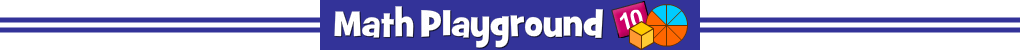Choose the largest starting number.
Place the puzzle piece on the missing number in the grid.
.Watch as the mystery picture appears.
Puzzle Pics Subtraction Facts - Learning Connections
Common Core Connection for Grades 1 and 2
Apply properties of operations as strategies to add and subtract.
Understand subtraction as an unknown-addend problem.
Relate counting to addition and subtraction.
Fluently add and subtract within 20 using mental strategies.
Use strategies such as counting on, making ten, decomposing numbers and creating easier or known sums.
More Math Games to Play